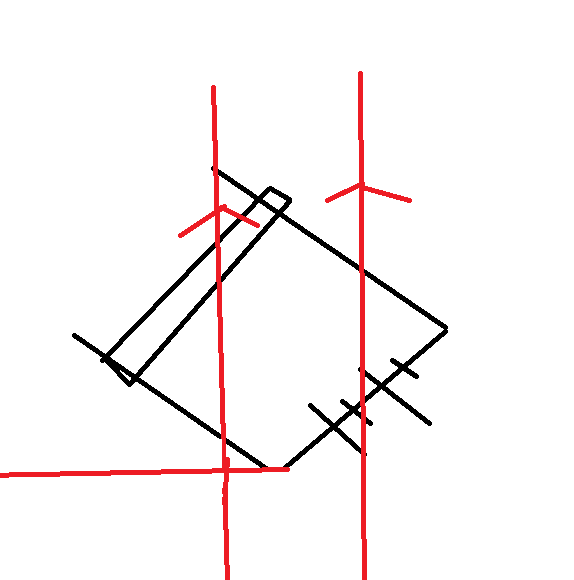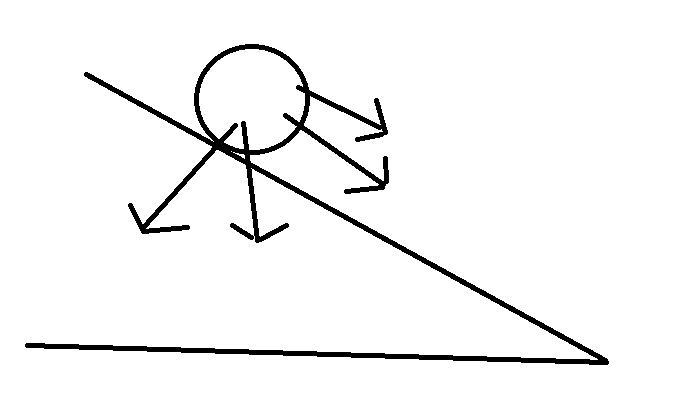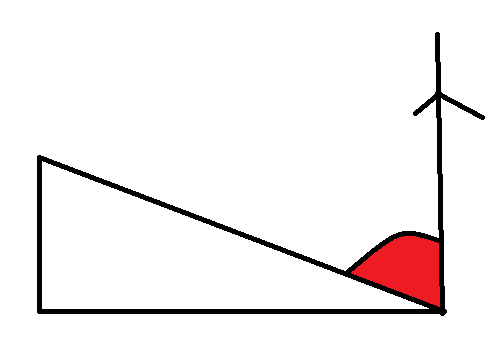# Rotating cylinder rolling without slipping in a B field

Homework Statement:
Cylinder length 1.2m, mass =0.1kg, radius 0.5cm is released from rest and roll down without slipping down 2 rails connected to the battery of 10V. Rails at angle of 40 degrees. B = 0.01T. The total resistance of rails and cylinder is 250 ohms. Calculate V after it has rolled down 0.45m
Relevant Equations:
-Firstly, I need to determine what the electric field is causing.
Using left hand rule, the force due to the field is acting down the slope.
Hence my FBD looks like:Where the two arrows pointing towards the right represent the force due to the field and weight of the cylinder.
Since :

##\epsilon = blv##
##I =\frac{\epsilon}{r} = \frac{blv}{R}##
Is it correct to say that:
##ma = mgsin\theta + F_{b} = mgsin\theta + bIl## ?

If so, what do I do next?

Rolling down 0.45m , does that mean I need to compute the time it takes to roll down using Pythagoras theorem? Or am I overthinking here?

Thank you

kuruman
Homework Helper
Gold Member
Firstly, I need to determine what the electric field is causing.
Using left hand rule, the force due to the field is acting down the slope.
Hence my FBD looks like:

View attachment 250424
Where the two arrows pointing towards the right represent the force due to the field and weight of the cylinder.
Please draw a better FBD and label all the forces. Do not include both the components of a force and the resultant.
Since :

##\epsilon = blv##
Doesn't the fact that the field is not at a right angle relative to the velocity make a difference?
##I =\frac{\epsilon}{r} = \frac{blv}{R}##
Is it correct to say that:
##ma = mgsin\theta + F_{b} = mgsin\theta + bIl## ?
No, it is not correct. If the cylinder rolls without slipping, there is an additional force acting on it. What is it?
If so, what do I do next?
You need to write an additional equation expressing Newton's 2nd law for rotations and combine that with the correct equation for the translation down the incline to find the linear acceleration. You also need to figure out the correct direction for the magnetic force on the cylinder.

I note that is problem is ill posed. As the cylinder rolls down, the amount of rail that is part of the circuit becomes shorter and hence the contribution of the rail to the resistance decreases. Usually, the rails have zero resistance and either the cylinder has resistance R or the cylider also has zero resistance but the rails are bridged with resistor R.

TSny
Homework Helper
Gold Member
Will the magnetic force on the cylinder have any appreciable effect on the motion of the cylinder? Compare the numerical values of ##mg## and ##bIl## for a current due to the 10 Volts and 250 Ohms.

Please draw a better FBD and label all the forces. Do not include both the components of a force and the resultant.
Do you mean by this?Doesn't the fact that the field is not at a right angle relative to the velocity make a difference?

In this case is the EMF:

##\epsilon = BLVsin\theta##
whereby ##\theta## = 90-40 =50 degrees?

No, it is not correct. If the cylinder rolls without slipping, there is an additional force acting on it. What is it?

That will be friction, as stated in my updated FBD.

You need to write an additional equation expressing Newton's 2nd law for rotations and combine that with the correct equation for the translation down the incline to find the linear acceleration. You also need to figure out the correct direction for the magnetic force on the cylinder.

I note that is problem is ill posed. As the cylinder rolls down, the amount of rail that is part of the circuit becomes shorter and hence the contribution of the rail to the resistance decreases. Usually, the rails have zero resistance and either the cylinder has resistance R or the cylider also has zero resistance but the rails are bridged with resistor R.

So combined with my updated existing equation where:

##ma = mgsin\theta + F_{b} - f_{s} = mgsin\theta + BIL -\mu_{k} (mgcos\theta)##

Finding an equation for rotation:

##\tau = I\alpha = \mu_{k}(mgcos\theta)(0.5cm)##

So am I supposed to find out the rotational acceleration? If so, what is the inertia?

•Delta2
kuruman
Homework Helper
Gold Member
Do you mean by this?

View attachment 250465
Yes, something like this. The direction of Fb is incorrect. What do you need to do to find the correct direction?
In this case is the EMF:
##\epsilon = BLVsin\theta##
whereby ##\theta## = 90-40 =50 degrees?
In a steady, uniform field, the magnitude of the induced emf is given by ##\epsilon=\dfrac{d}{dt}(\vec B\cdot\hat n~A)##, where ##\hat n## is the normal to the rectangular loop the area ##A## of the loop that is shrinking. Figure it out from there.
That will be friction, as stated in my updated FBD.
Yes, there is friction, but what kind, static or kinetic? If kinetic, it is opposite to the velocity; if static, it is in whatever direction is appropriate to provide the observed acceleration.
So combined with my updated existing equation where:
##ma = mgsin\theta + F_{b} - f_{s} = mgsin\theta + BIL -\mu_{k} (mgcos\theta)##
Even though you use ##f_s## to denote static friction you set it equal to the expression of kinetic friction ##\mu_k N##. You cannot do this.
Finding an equation for rotation:
##\tau = I\alpha = \mu_{k}(mgcos\theta)(0.5cm)##
So am I supposed to find out the rotational acceleration? If so, what is the inertia?
Yes, find the rotational acceleration and relate it to the linear acceleration. For this particular problem, it is easier to calculate torques about the point of contact. Even though the number of torques is more than one about that point, the torque provided by friction is zero so you don't need to know what it is and the torque equation is sufficient to give you the acceleration. You can look up the moment of inertia of a cylinder about its axis on the internet. Should you choose to write the torque equation about the point of contact as I suggested, don't forget to use the parallel axis theorem to find the moment of inertia about that point.

A final note: To avoid confusion, don't use ##I## for both current and moment of inertia. By the way, what is an expression for the current through the cylinder?

Yes, something like this. The direction of Fb is incorrect. What do you need to do to find the correct direction?
Oh, I figured that my left-hand rule was wrong because I misread how current is travelled.

I figured out that the force caused by magnetic field is: F=BIL(sin50) since the angle between current and magnetic field is 90-40.

Upon further asking my teacher for hints, he stated that with this force I can simply find the acceleration by diving by mass since F=ma.

The question is what about friction and mgsin theta? Why is it applicable to ignore in this case?

Thanks

kuruman
Homework Helper
Gold Member
Oh, I figured that my left-hand rule was wrong because I misread how current is travelled.
It's a right hand rule. The left hand rule gives you the opposite direction.
I figured out that the force caused by magnetic field is: F=BIL(sin50) since the angle between current and magnetic field is 90-40.
You figured out incorrectly. Look at your drawing. The fact that the cylinder lies on an incline is irrelevant.
Upon further asking my teacher for hints, he stated that with this force I can simply find the acceleration by diving by mass since F=ma.
Your teacher's suggestion is to the point but not very useful. You can always find the acceleration by dividing the net force Fnet by the mass. The real question is how to find an expression for Fnet. To do that (a) draw a complete free body diagram; (b) find expressions for all the forces in the diagram in terms of symbols representing the given quantities and (c) add all these forces as vectors to find the net force.
The question is what about friction and mgsin theta? Why is it applicable to ignore in this case?
Friction is one of the forces acting on the cylinder and ##mg\sin\theta## is the component of the weight parallel to the incline. Neither is (or should be) ignored.

The two equations you must express in terms of the given quantities symbolically are$$\vec F_{net}=m\vec a_{cm}~;~~\vec {\tau}_{net}=I\vec {\alpha}$$Because the cylinder rolls without slipping, you can relate the magnitude of the acceleration of the center of mass to the magnitude of the angular acceleration by ## a_{cm}=\alpha R##, where ##R## is the radius of the cylinder, not the resistance.

Perhaps you should first solve this problem in zero magnetic field with the cylinder just rolling down the incline due to the effect of gravity alone. That will show you how the two equations can be brought together to get the acceleration. Once you understand that, then you can add the magnetic field. As @TSny suggested in #3, you may find that given the numbers in this problem, the effect of having the field on is just as negligible as air resistance. IMO the pedagogical value of this problem lies in solving it symbolically as given.

As @TSny suggested in #3, you may find that given the numbers in this problem, the effect of having the field on is just as negligible as air resistance. IMO the pedagogical value of this problem lies in solving it symbolically as given.
Is that why the resulting ##F_{net}## is only due to the force made by the field as a result?

The fact that the cylinder lies on an incline is irrelevant.Isn't the red angle supposed to be angle between the field and the velocity (hence 90-40)?

kuruman